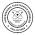### OHM’s LAW EXERCISE2

Exercise2

1.         Suppose that the dc generator in Figure 1 produces 10 V and the potentiometer is set to a value of 10 ohms. What is the current?

2.         Imagine that dc generator in Figure 1 produces 100 V and the potentiometer is set to 10 kiloohms. What is the current?

3.         Suppose that dc generator in Figure 1 is set to provide 88.5 V, and the potentiometer is set to 477 Megaohms. What is the current?

4.         Suppose the potentiometer in Figure 1 is set to 100 ohms, and the measured current is 10 mA. What is the dc voltage?

5.         Adjust the potentiometer in Figure 1 to a value of 157 kiloohms, and suppose the current reading is 17.0 mA. What is the voltage of the source?

6.         Suppose you set the potentiometer in Figure 1 so that the meter reads 1.445 A, and you observe that the potentiometer scale shows 99 ohms. What is the voltage?

7.         If the voltmeter in Figure 1 reads 24 V and the ammeter shows 3.0 A, what is the resistance of the potentiometer?

8.         What is the value of the resistance in Figure 1 if the current is 18 mA and the voltage is 229 mV?

9.         Suppose the ammeter in Figure 1 reads 52 uA and the voltmeter indicates 2.33 kV. What is the resistance?

10.       Suppose that the voltmeter in Figure 1 reads 12 V and the ammeter shows 50 mA. What is the power dissipated by the potentiometer?

11.       If the resistance in the circuit of Figure 1 is 999 ohms and the voltage source delivers 3 V, what is the power dissipated by the potentiometer?

12.       Suppose the resistance in Figure 1 is 47 kiloohms and the current is 680 mA. What is the power dissipated by the potentiometer?

13.       How much voltage would be necessary to drive 680 mA through a resistance of 47 kiloohms?

14.       Consider five resistors in parallel. Call them R1 through R5. Let the resistance values be as follows: R1 = 100 ohms, R2 = 200 ohms, R3 = 300 ohms, R4 = 400 ohms, and R5 = 500 ohms. What is the total resistance?

15.       cont....  What is the total conductance?

1.1.2.1.